### 14.6.3.2 Determining the allowable accelerations

The actions in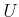that cause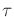to be followed can now be characterized. An action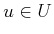followsif and only if every equation of the form (14.39) is satisfied. If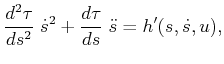for allfromto, thensuch equations exist. Suppose that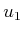is chosen, and the first equation is solved for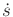. The required values of the remaining action variables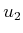,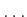,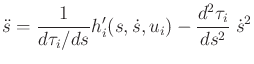can be obtained by substituting the determinedvalue into the remaining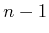equations. This means that the actions that followare at most a one-dimensional subset of.

If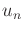for some, then following the path requires that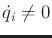. Instead of (14.39), the constraint is that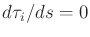. Example 14.6 will provide a simple illustration of this. Iffor all, then the configuration is not allowed to change. This occurs in the degenerate (and useless) case in whichis a constant function.

In many cases, a value ofdoes not exist that satisfies all of the constraint equations. This means that the path cannot be followed at that particular state. Such states should be removed, if possible, by defining phase constraints on. By a poor choice of pathviolating such a phase constraint may be unavoidable. There may exist some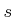for which nocan follow, regardless of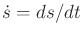.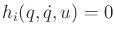Even if a state trajectory may be optimal in some sense, its quality ultimately depends on the given path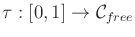. Consider the path shown in Figure 14.26. At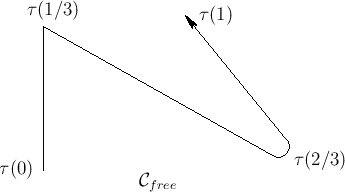, a corner'' is reached. This violates the differentiability assumption and would require infinite acceleration to traverse while remaining on. For some models, it may be possible to stop atand then start again. For example, imagine a floating particle in the plane. It can be decelerated to rest exactly atand then started in a new direction to exactly follow the curve. This assumes that the particle is fully actuated. If there are nonholonomic constraints on, as in the case of the Dubins car, then the given path must at least satisfy them before accelerations can be considered. The solution in this case depends on the existence of decoupling vector fields [157,224].

It is generally preferable to round off any corners that might have been produced by a motion planning algorithm in constructing. This helps, but it still does not completely resolve the issue. The portion of the path around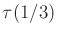is not desirable because of high curvature. At a fixed speed, larger accelerations are generally needed to follow sharp turns. The speed may have to be decreased simply becausecarelessly requires sharp turns in. Imagine developing an autonomous double-decker tour bus. It is clear that following the curve aroundmay cause the bus to topple at high speeds. The bus will have to slow down because it is a slave to the particular choice of.

Steven M LaValle 2012-04-20